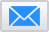To: (Separate email addresses with commas)
Message: (Optional)

## Thanks!

•# Easy Mental Math For Pilots

Boldmethod

If you're like us, you probably don't consider yourself a math expert. Here are a few easy tips and tricks you can use to make mental math in the cockpit a little easier...

## Descent Planning Mental Math

• Step 1) How much altitude do I need to lose?
• Step 2) How much time to reach the fix?
• Step 3) Altitude to lose / Time = FPM Descent Rate

If you're supposed to answer a mental math question for an interview or test, stick to whole, even rounded, numbers. You're not expected to be a flight computer!

Step 1: How much altitude do I need to lose? When you're doing this, stick to rounded, whole numbers. Do you need to lose 5, 10, 15, or 20 thousand feet? That's the altitude value you'll want to keep in mind.

If you need to lose 3,800 feet, round up to 4,000. In most cases, that will make your mental math a lot easier. More on that in a bit...

Step 2: How much time to reach the fix? This is a two-step process. First, you'll figure out how many miles-per-minute (MPM) you're flying.

Think in multiples of 60.

60 knots is 1 mile per minute. 120 knots is 2 MPM. Double that (240 knots), and you're going 4 MPM. If the numbers seem too big to work with, take the zero away and make the values 6, 12, or 24.

For example, let's say you're going 180 knots. 6 goes into 18 three times, so that's 3 MPM.

• 60 knots = 1 MPM
• 90 knots = 1.5 MPM
• 120 knots = 2 MPM
• 150 knots = 2.5 MPM
• 180 knots = 3 MPM

Remember, these speeds are ground speed. When it comes to figuring out your MPM, ground speed is the only speed that matters.

Now that you've got the "miles per minute value", let's look at how far you need to fly.

If you need to fly 20 miles, and you're flying 2 MPM, it'll take you 10 minutes to reach the fix (20 miles / 2 MPM = 10 minutes).

Step 3: Altitude to lose / Time = FPM Descent Rate... Here's an example of a calculated descent rate:

## Other Uses For The 60-1 Rule

The basic rule says "at a 1 degree slope (or 1 degree on your attitude indicator or HSI), it's going to be 60 units horizontally for 1 unit vertically." But how else does this apply to your flying?

## VOR Courses

If you're flying towards a VOR and you're 1 degree off course at 60 miles, you're 1 mile off track. If you're 2 degrees off track at 60 miles, you'd be 2 miles off track. At 30 miles and 1 degree of deviation, you're 1/2 mile of track. And at 15 miles and 1 degree of deviation, you're only 1/4 mile off track.

## DME Arcs

This math can help with flying arcs too. A question you might get during an airline interview is, "how long is this arc segment?"

Let's take a look at this unusual DME arcing approach.

In an arc is 14.7 DME from the VOR, let's call that 15 miles. At 15 miles, every degree flown around the arc takes 1/4 mile. If the arc spans from radial 334 to 060 degrees, that's 86 degrees.

Since every degree of the arc is .25 miles, what's a quarter of 86? Without a calculator, you probably can't work that out in your head.

But, what's a quarter of 80? It'd be 20! (80 / 4 = 20) Since we took 6 off of 86 degrees to make the math easy, let's work on that remaining 6. One-quarter of 6 is 1.5 miles.

So in this quesiton the arc segment is 21.5 miles long (20 + 1.5 = 21.5 miles).

## Descent Angles

If you know your flight path angle (FPA), which you'll often find in modern flight decks, the 60-1 rule can make mental math descent planning easy.

For every 1 degree of descent angle, you'll descent 100 feet for every 1 mile you fly.

For example, if you're descending at a 3 degree angle for 3 miles, you'll descend 900 feet.

What else do you use mental math for in the cockpit? Tell us in the comments below.### Colin Cutler

Colin is a Boldmethod co-founder, pilot and graphic artist. He's been a flight instructor at the University of North Dakota, an airline pilot on the CRJ-200, and has directed development of numerous commercial and military training systems. You can reach him at colin@boldmethod.com.

Images Courtesy:

## Latest Stories